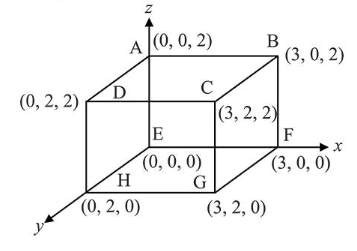# An electric fieldQuestion:

An electric field $\overrightarrow{\mathrm{E}}=4 x \hat{i}-\left(y^{2}+1\right) \hat{j} \mathrm{~N} / \mathrm{C}$ passes through the box shown in figure. The flux of the electric field through surfaces $\mathrm{ABCD}$ and BCGF are marked as $\phi_{1}$ and $\phi_{11}$ respectively. The difference between $\left(\phi_{1}-\phi_{11}\right)$ is (in $\mathrm{Nm}^{2} / \mathrm{C}$ )_________Solution:

$(-48)$

Flux of electric field $\vec{E}$ through any area $\vec{A}$ is defined as

$\phi=\int E \cdot A \cos \theta$

Here, $\theta=$ angle between electric field and area vector of a surface

For surface $A B C D$ Angle, $\theta=90^{\circ}$

$\therefore \quad \phi_{1}=\int E \cdot A \cos 90^{\circ}=0$

For surface $B C G F \phi_{n}=\int \vec{E} \cdot \overrightarrow{d A}$

$\therefore \phi_{11}=\left[4 \times \hat{i}-\left(y^{2}+1\right) \hat{j}\right] \cdot 4 \hat{i}=16 x$

$\phi_{11}=48 \frac{\mathrm{Nm}^{2}}{C}$

$\phi_{1}-\phi_{11}=-48$